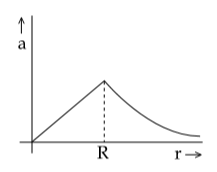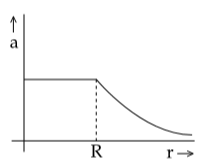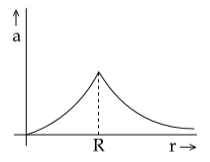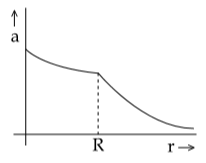Q

# Solve! - Gravitation - JEE Main

The mass density of a spherical body is  given by ρ (r)=for r ≤ R and ρ (r)=0 for r > R, where r is the distance from the centre. The correct graph that describes qualitatively the acceleration, a, of a test particle as a function of r is

• Option 1)• Option 2)• Option 3)• Option 4)151 Views

As we learnt in

Gravitational field Intensity -

- wherein

Given that  of spherical body

inside the surface of sphere intensity

or

Option 1)Incorrect

Option 2)Correct

Option 3)Incorrect

Option 4)Incorrect

Exams
Articles
Questions